# SQL Date Greater Than Query

In today’s tutorial, we are going to discuss and execute the SQL date greater than query in SQL, let’s start with the introduction first.

## Introduction

The greater than(>) operator in SQL is used in a condition where we have to compare 2 expressions or values.

For better understanding check out the queries below.

## SQL Date Greater Than Queries & Examples

First of all, we will create the database and then we will create a table.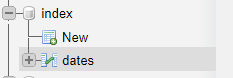We created a database and then named it “index” and the table named “dates”, you can use any name.

Our table looks like this.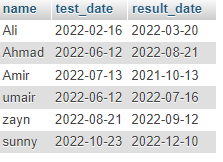After creating the table use the following query to find the greater date.

``SELECT * FROM dates WHERE result_date > '2022-01-25'``

The above query states that select all columns from the table “dates” where (using condition)result_date is greater than “2022-01-25”.

This query will return those dates that are greater than the “2022-01-25” which means those dates will be shown which are placed after the “2022-01-25” let’s check the output.

OutputAs you can see in the output above it returned all those dates that are greater than “2022-01-25”.

We have multiple ways to write this query and check out the next.

``SELECT test_date, result_date FROM dates WHERE result_date > test_date``

The above query states that select test_date from the table “dates” where result_date is greater than the test_date, this query will return all result dates.

Output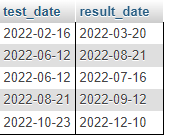As you can see the output above it returned all the result dates that are greater than the test dates according to the query.

Let’s see the next query.

``````SELECT test_date, result_date FROM dates WHERE result_date > CURRENT_DATE();
``````

The above query states that select the test_date column and result date column From the table “dates” where(using condition) result_date is greater than the present or current date(current date function).

Let’s see the results below.

Output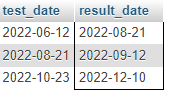As you can see in the above output the query returned the result dates greater than the present day date.

Our next query is.

``SELECT * FROM dates WHERE DATE(result_date) > CURDATE()``

The above query states that select all columns from the table “dates” where (using condition) the result date is greater than the current date, check out the result below.

Output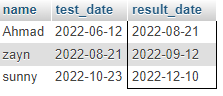As you can see the output above returned the same output as the previous query returned.

We can use more functions in the query, see our next query below.

``SELECT * FROM dates WHERE DATE(result_date) > SUBDATE(CURRENT_DATE, 1)``

It will select all columns from the table “dates” where(condition) result_date will be greater than the current date.

We used the date and SUBDATE function in the where clause so these functions can subtract the dates that are less than the current date, in the SUBDATE function we passed the current date and 1 as a parameter.

See the results below.

Output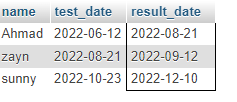As you can see above output returned the same output as the previous query returned.

Let’s move forward to our last query.

``SELECT * FROM dates WHERE DATE(result_date) > ADDDATE(CURDATE(), 1)``

It will select all columns from the table “dates” where(condition) result_date will be greater than the current date.

We used the date and ADDDATE function in the where clause and passed the current date and 1 as a parameter in the function.

Let’s check the results:

Output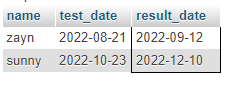As you can see the output above it displayed 2 records these are the dates greater than the current date.

## Conclusion

In this tutorial we talked about the SQL date greater than Query, we discussed a short introduction to the greater than operator first then we saw the queries to find the dates greater than current dates.

We used different functions as well with the greater than operator that also returned the same results.

That’s all for today hope you like the tutorial if you have any queries regarding this tutorial so feel free to contact us below. Thanks for reading.

Suggested Article: